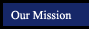Geometry Postulates and Theorems
textbook tutorials - definitions - constructions - postulates and theorems - internet activities and resources
 symmetry and similarity points, lines and planes parallel lines triangles quadrilaterals polygons circles 3-Dimensional Geometry
Triangle Theorems
Right Triangle Theorems
Triangle Inequality Theorem:
The sum of the lengths of any two sides of a triangle is greater than the length of the third side.
If the alttitude is drawn to the hypotenuse of a right triangle,
then the two triangles formed are similar to the original triangle and to each other.
One side of a triangle is longer than a second side if and only if
the angle opposite the first side is larger then the angle opposite the second side.
If the altitude is drawn to the hypotenuse of a right triangle,
then the length of the altitiude is the geometric mean of the lengths of the segments of the hypothenuse.
Exterior Angle of a Triangle Theorem
The measure of an exterior angle of a triangle is equal to the sum of the meaures of the two interior angles not adjacent to it.
If the altitude is drawn to the hypotenuse of a right triangle,
then the length of each leg is the geometric mean of the lengths of the hypotenuse and the segment of the hypotenuse adjacent to that leg.

SSS Theorem
If three sides of one triangle are congruent to the corresponding sides of another triangle, then the triangles are congruent.

In a 45-45-90 triangle,
the hyptenuse is square root of 2 times the length of each leg.
SAS Theorem
If two sides and their included angle are congruent to the corresponding sides of another triangle, then the triangles are congruent.
In a 30-60-90 triangle
the length of the shorter leg and the length of the longer leg is square root of times the length of the shorter leg.
AAS Theorem
If two angles and a non-included side of one triangle are congruent to the corresponding parts of another trianlge
then the triangles are congruent.
The Pythagorean Theorem
In a right triangle the square of the length of the hypotenuse is equal to the sum of the squares of the lengths of the legs.
HL Theorem
If the hypotenuse and a leg of one right triangle are congruent to the corresponding parts of another right triangle
then the triangles are congruent.
Converse of the Pythagorean Theorem
If a, b, and c are the lengthsof the sides of a triangle and a + b = c,
then the trianlge is a right triangle.
If a point is on the perpendicular bisector of a segment
then the point is equaldistant from the endpoints of the segments.

The Isosceles Triangle Theorem
If two sides of a triangle are congruent
then the angles opposite the sides are congruent.

The Converse of the Isosceles Triangle Theorem
If two angles of a triangle are congruent,
then the sides opposite the angles are congruent.